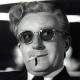A proof of the famous Cantor TheoremNeil L. is not a computer scientist–he is a Leprechaun. Since today is St. Patrick’s Day it seems right to talk about him again. As long as you look at him he cannot escape, but the moment you look away, he vanishes.

Magic.

I want to present a proof of Cantor’s famous Theorem: the set of all infinite sequences of${0}$${1}$‘s is not countable. Let$\displaystyle s^{1}, s^{2}, \dots, s^{n}, \dots$

be a sequence of infinite sequences. Each${s^{i}}$ is an infinite sequence; for example,$\displaystyle 00000 \dots 0000 \dots$

could be${s^{1}}$.

The plan is to use the probabilistic method. Let${X_{1},X_{2},\dots}$ be an infinite sequence of independent indicator random variables that are${1}$ with probability${1/2}$. Define another sequence of indicator random variables${Y_{1},Y_{2},\dots}$ as follows:${Y_{i}}$ is${1}$ if and only if$\displaystyle X_{1},\dots,X_{i+1} = s^{i}(1),\dots,s^{i}(i+1).$

Then, the expectation${E[Y_{i}]}$ is equal to${2^{-(i+1)}}$. Consider next the infinite sum,$\displaystyle A = \sum_{i=1}^{\infty} Y_{i}.$

By linearity of expectation it follows that$\displaystyle E[A] = 1/4 + 1/8 + \dots = 1/2.$

Thus, there is a sample point so that all the${Y_{i}}$‘s are${0}$. But, this point must be different from each sequence${s^{i}(1),s^{i}(2),\dots}$ For suppose that${x_{1},x_{2},\dots}$ is the sample point. Then, since all${Y_{i}=0}$ it must follow that$\displaystyle x_{1},\dots,x_{i+1} \neq s^{i}(1),\dots,s^{i}(i+1).$

Open Problems

Have a happy St. Patrick’s Day. By the way is this proof correct or has Neil tricked us?

1.March 17, 2010 9:40 am

Did you forget to show that the Yi are independent?

•March 17, 2010 11:51 am

Nemo,

Love pic. No. They are not independent. But E[A+B] = E[A] + E[B] whether independent or not. That is the beauty of expectation.

•March 17, 2010 12:03 pm

Whoops; never mind. Linearity of expectation holds for non-independent events.

But something is still bothering me about that infinite sum. For some X, it diverges…

2.March 17, 2010 10:57 am

Impressive post this emerald day.

3.March 17, 2010 11:45 am

I’m not sure I would be convinced by this argument if I didn’t already know Cantor’s Theorem.

The problem is that you don’t reprove all the theorems of probability theory that you use. Without that, how do I know that none of the proofs depend on the uncountably of the reals?

Using probability theory to prove Cantor’s Theorem is like turning wine into water. It may be an interesting trick, but didn’t you need water to grow the grapes?

4.March 17, 2010 12:32 pm

I’m mostly with Ralph Hartley, but to me the issues are more of “Reverse Mathematics”—any followers of Harvey Friedman at the ASL meeting? The question is whether the deduction of a sample point such that all Y_i’s are 0 depends on infinitistic facts about Lebesgue measure, which in turn depend on Cantor. The argument is based on the binary tree of sequences rather than the reals, so the basic open sets (corresponding to each node) are truly finitistic. The question IMHO is whether that’s enough of a foundation for proving that the leprechaun cannot have led us into a “Tarski World” of non-measurable sets that can make a part seem to equal the whole.

•5.